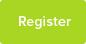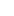# Tutor Hunt Questions

what is the pH of an acetate buffer prepared from 100mL of 0.1mol/L acetic acid solution and 75mL of a 0.2mol/L sodium acetate solution? the pKa of acetic acid is 4.76?
3 years ago

Please enter your response to the question below. The student will get a notification as soon your response has been approved by our moderation team.

Ka = [CH3CO2-]*[H+] / [CH3CO2H]
This is equivalent to [H+] = Ka *[CH3CO2H]/[CH3CO2-] equation 1

pH = -log [H+]

pH = -log (Ka*[CH3CO2H]/[CH3CO2-])
pH= -log (Ka) - log([CH3CO2H]/[CH3CO2-])
pH = pKa + log([CH3CO2-]/[CH3CO2H])equation2
as log(a*b) = log(a) + log(b) and log(a/b) = -log(b/a)

mole (CH3CO2-) = 0.2 x 75/1000 = 0.015
mole (CH3CO2H) = 1 x 100/1000 = 0.1
V = 100 + 75 = 175 mL
[conc] = mol/V

pH = 4.76 + log (0.015 / 0.1), V cancels out
pH = 4.76 + log(0.15)
pH = 3.9

Recommended method if not comfortable using equation 2:
pH = pKa + log[base]/[acid]

Calculate [H+] using eq 1 and Ka = 10^-pKa

[H+] = 10^-4.76 x 0.1 / 0.015, V cancel out
[H+] = 1.16 x 10^-4 mol/L
pH = -log[H+] = 3.9

:)
Answered by Magalie | 3 years ago
pH = pKa + lg 1.5